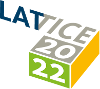#The 39th International Symposium on Lattice Field Theory (Lattice 2022)

Aug 8 – 13, 2022
Hörsaalzentrum Poppelsdorf
Europe/Berlin timezone

## NLO perturbative effects in QCD sum-rule analyses of light tetraquark systems: \\A Case Study in the Scalar-Isoscalar Channel

Aug 9, 2022, 7:00 PM
1h
Poster Presentation Hadron Spectroscopy and Interactions

### Speaker

Barbara Cid-Mora (GSI Helmholtzzentrum für Schwerionenforschung GmbH)

### Description

QCD sum-rule mass predictions for tetraquark states provide insights on the interpretations and internal structure of experimentally-observed exotic mesons. However, the overwhelming majority of tetraquark QCD sum-rule analyses have been performed at leading order (LO), which raises questions about the underlying theoretical uncertainties from higher-loop corrections. The impact of next-to-leading order (NLO) perturbative effects are systematically examined in scalar ($J^{PC}=0^{++}$) isoscalar light-quark tetraquark systems where comprehensive LO sum-rule analyses have been performed and NLO perturbative corrections to the correlators have previously been calculated. Using the scalar-isoscalar state as a detailed case study to illustrate the differences between LO and NLO analyses, it is shown that NLO effects in individual Laplace sum-rules are numerically significant and have an important role in improving the reliability of the sum-rule analyses by widening the Borel window. However, ratios of sum-rules are found to be less sensitive to NLO effects with the additional advantage of cancelling the anomalous dimension that emerges from the NLO corrections. NLO mass predictions based on these sum-rule ratios are thus remarkably robust despite the slow perturbative convergence of the underlying correlator. The mass prediction $0.52\,\text{GeV}< m_{\sigma}<0.69\,\text{GeV}$ for the lightest scalar-isoscalar $\sigma$ state are in good agreement with the four-quark interpretation of the $f_0(500)$, and the relative coupling strengths of the $f_{0}(980)$ and $f_0(500)$ to the tetraquark current agree with the pattern found in chiral Lagrangian analyses. Effects of the $\sigma$ resonance width are studied for a different models, including resonance shapes inspired by chiral Lagranians.

### Primary author

Barbara Cid-Mora (GSI Helmholtzzentrum für Schwerionenforschung GmbH)

### Co-author

Prof. Tom Steele (University of Saskatchewan)